# Scattered space

Scattered spaces have their origin in Cantors investigations into the uniqueness of trigonometric series. His theorem reads (in modern terminology): If the partial sums of a trigonometric series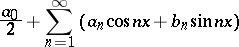converge to zero except possibly on a set of points of finite scattered height, then all coefficients of the series must be zero.

Scattered spaces and their scattered height are defined as follows. One first defines for any spacea transfinite sequence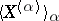of subspaces: Let, for any ordinal, let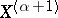be the derived set of, and ifis a limit ordinal, put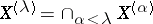.

There is a first ordinal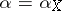for which. If this derived set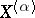is empty, one callsa scattered space and the ordinal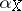its scattered height.

Ifis compact, then it is readily seen thatmust be a successor ordinal, say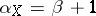. The set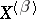is finite. It is a classical result of S. Mazurkiewicz and J. Sierpiński that a countable compact scattered spaceis determined completely by the ordinaland the numberof points in:is homeomorphic to the set of ordinal numbers less than or equal to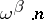.

Compact scattered spaces correspond, via Stone duality, to the so-called superatomic Boolean algebras; these are defined to be those algebras for which every (non-trivial) homomorphic image has an atom. Because of this duality one may say that the structure of compact scattered spaces is understood best.

An important family of scattered spaces (of height) is constructed as follows: Take an infinite setand a familyof countably-infinite subsets that is almost disjoint, i.e., if, then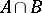is finite. The union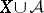is topologized by declaringto be an open discrete subspace and giving an elementofbasic neighbourhoods of the form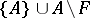, whereis a finite subset of. By varying the familyone can obtain various interesting examples of topological spaces, for example, in this way one can make a pseudo-compact space that is not countably compact (cf. Countably-compact space).